Question

1. Consider flipping a fair coin three times and observe whether it lands heads up or...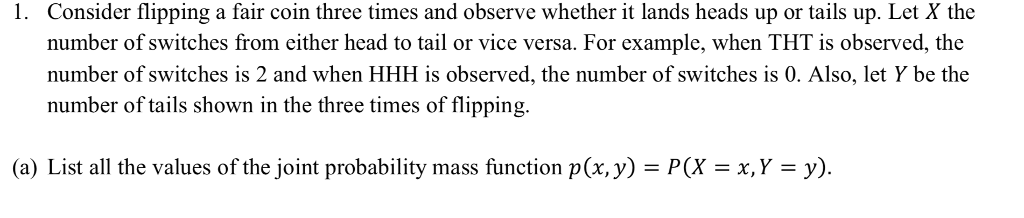1. Consider flipping a fair coin three times and observe whether it lands heads up or tails up. Let X the number of switches from either head to tail or vice versa. For example, when THT is observed, the number of switches is 2 and when HHH is observed, the number of switches is 0. Also, let Y be the number of tails shown in the three times of fipping. (a) List all the values of the joint probability mass function p(x,y) = P(X = x,Y = y).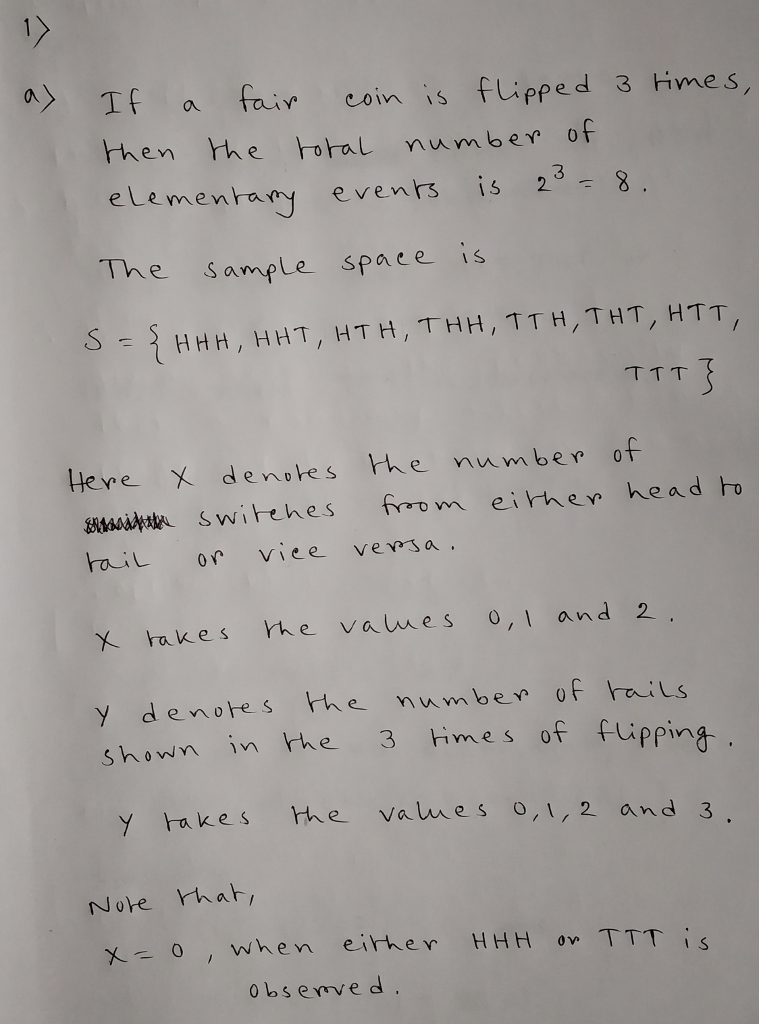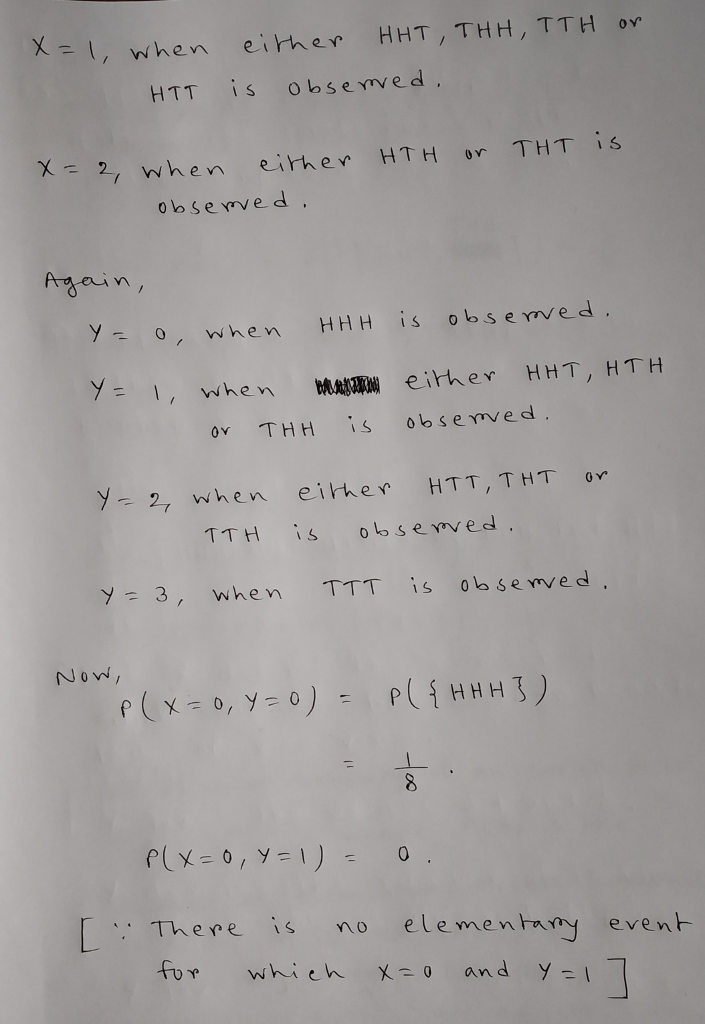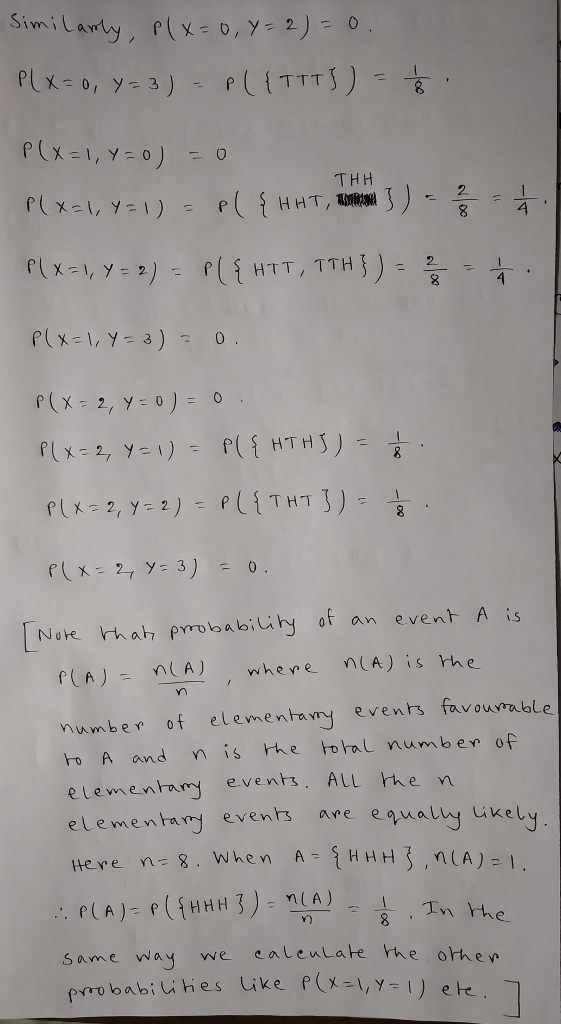Earn Coins

Coins can be redeemed for fabulous gifts.

Similar Homework Help Questions
• Question is are X and Y independent? Why? 1. Consider flipping a fair coin three times...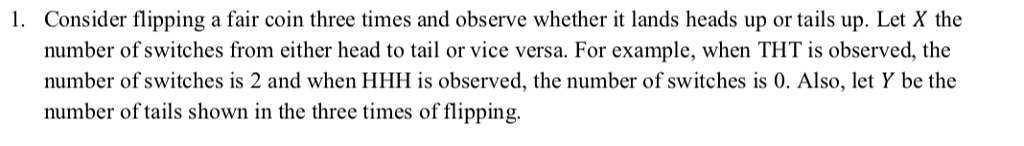Question is are X and Y independent? Why? 1. Consider flipping a fair coin three times and observe whether it lands heads up or tails up. Let X the number of switches from either head to tail or vice versa. For example, when THT is observed, the number of switches is 2 and when HHH is observed, the number of switches is 0. Also, let Y be the number of tails shown in the three times of flipping.

• You toss a penny and observe whether it lands heads up or tails up. Suppose the...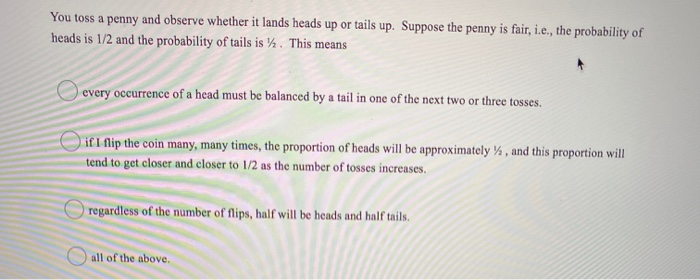You toss a penny and observe whether it lands heads up or tails up. Suppose the penny is fair, i.e., the probability of heads is 1/2 and the probability of tails is y. This means every occurrence of a head must be balanced by a tail in one of the next two or three tosses. if I flip the coin many, many times, the proportion of heads will be approximately %, and this proportion will tend to get closer and...

• 11. What are the possible combination outcomes when you toss a fair coin three times? (6.25...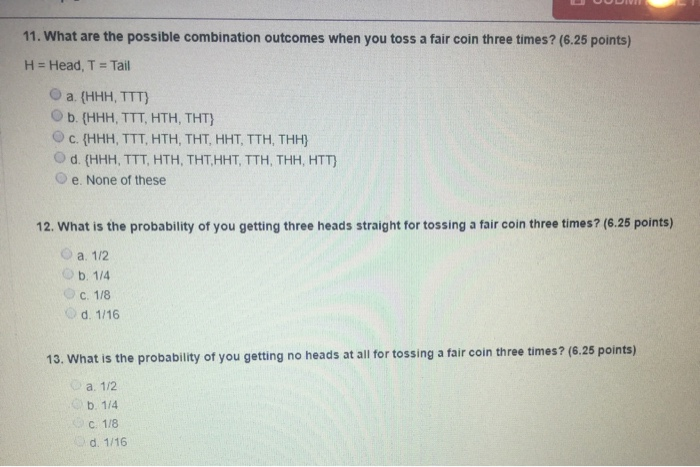11. What are the possible combination outcomes when you toss a fair coin three times? (6.25 points) H = Head, T = Tail a {HHH, TTT) Ob. (HHH, TTT, HTH, THT) c. {HHH, TTT, HTH, THT, HHT, TTH, THH) d. (HHH, TTT, HTH, THT, HHT, TTH, THH, HTT} e. None of these 12. What is the probability of you getting three heads straight for tossing a fair coin three times? (6.25 points) a. 1/2 OD. 1/4 C. 118 d. 1/16...

• A fair coin is tossed four times, and each time the coin lands heads up. If the ...continues

A fair coin is tossed four times, and each time the coin lands heads up. If the coin is then tossed 1996 more times, how many heads are most likely to appear forthese 1996 additional tosses?(a) 996(b) 998(c) 1000(d) 1996(e) None of the above. The answer is________________

• For a fair coin tossed three times, the eight possible simple events are HHH, HHT, HTH, THH, HTT, THT, TTH, TTT

For a fair coin tossed three times, the eight possible simple events are HHH, HHT, HTH, THH, HTT, THT, TTH, TTT. Let X = number of tails. Find the probability distribution for X by filling in the table showing each possible value of X along with the probability that value occurs. k=0,1,2,3 P(X=k)

• Assume that a coin is flipped where the probability of coin lands "Heads" is 0.49. The...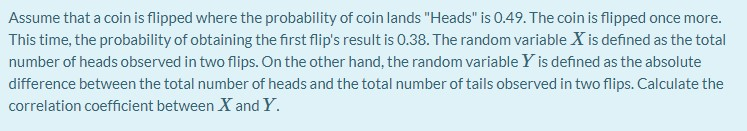Assume that a coin is flipped where the probability of coin lands "Heads" is 0.49. The coin is flipped once more. This time, the probability of obtaining the first flip's result is 0.38. The random variable X is defined as the total number of heads observed in two flips. On the other hand, the random variable Y is defined as the absolute difference between the total number of heads and the total number of tails observed in two flips. Calculate...

• The probability of observing HHH after flipping a fair coin three times equals 0.125 or 12.5...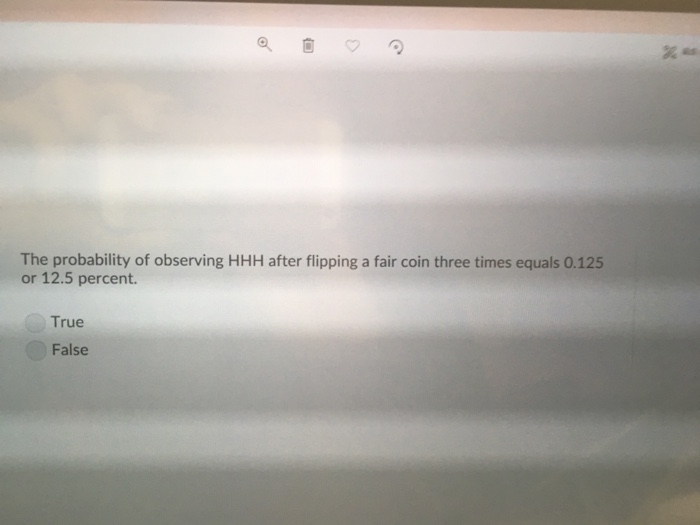The probability of observing HHH after flipping a fair coin three times equals 0.125 or 12.5 percent. True False

• 7.) Suppose that a fair coin is tossed 10 times and lands on heads exactly 2...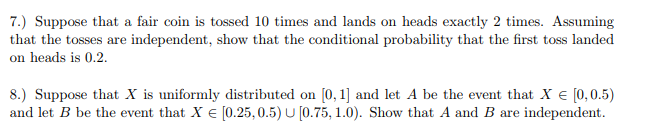7.) Suppose that a fair coin is tossed 10 times and lands on heads exactly 2 times. Assuming that the tosses are independent, show that the conditional probability that the first toss landed on heads is 0.2. 8.) Suppose that X is uniformly distributed on [0,1] and let A be the event that X є 10,05) and let B be the event that X e [0.25,0.5) U[0.75,1.0). Show that A and B are independent.

• 9) A fair coin is tossed n times, coming up Heads Nh times and Tails Nr...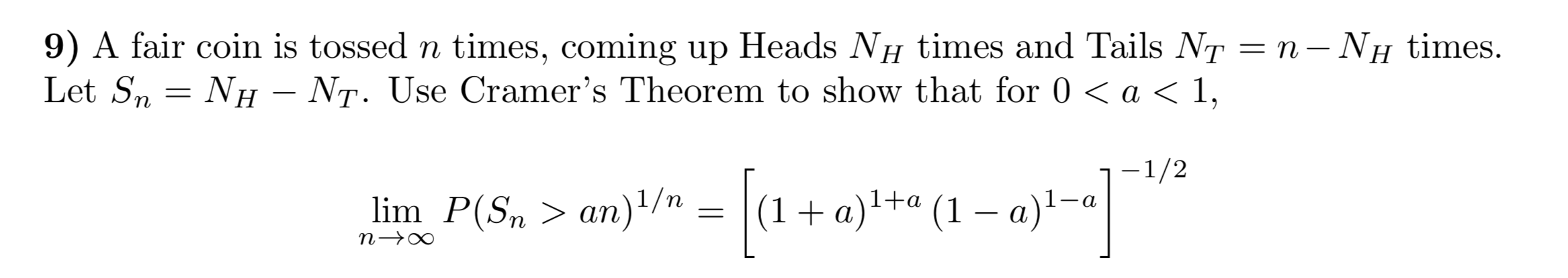9) A fair coin is tossed n times, coming up Heads Nh times and Tails Nr = n – Nh times. Let Sn = Nh – Nt. Use Cramer's Theorem to show that for 0 < a < 1, 1-1/2 lim n-> P(Sn. = ( + (1 - a)1-a

• What is the probability that a fair coin lands tails up ten times in a row?

What is the probability that a fair coin lands tails up ten times in a row?